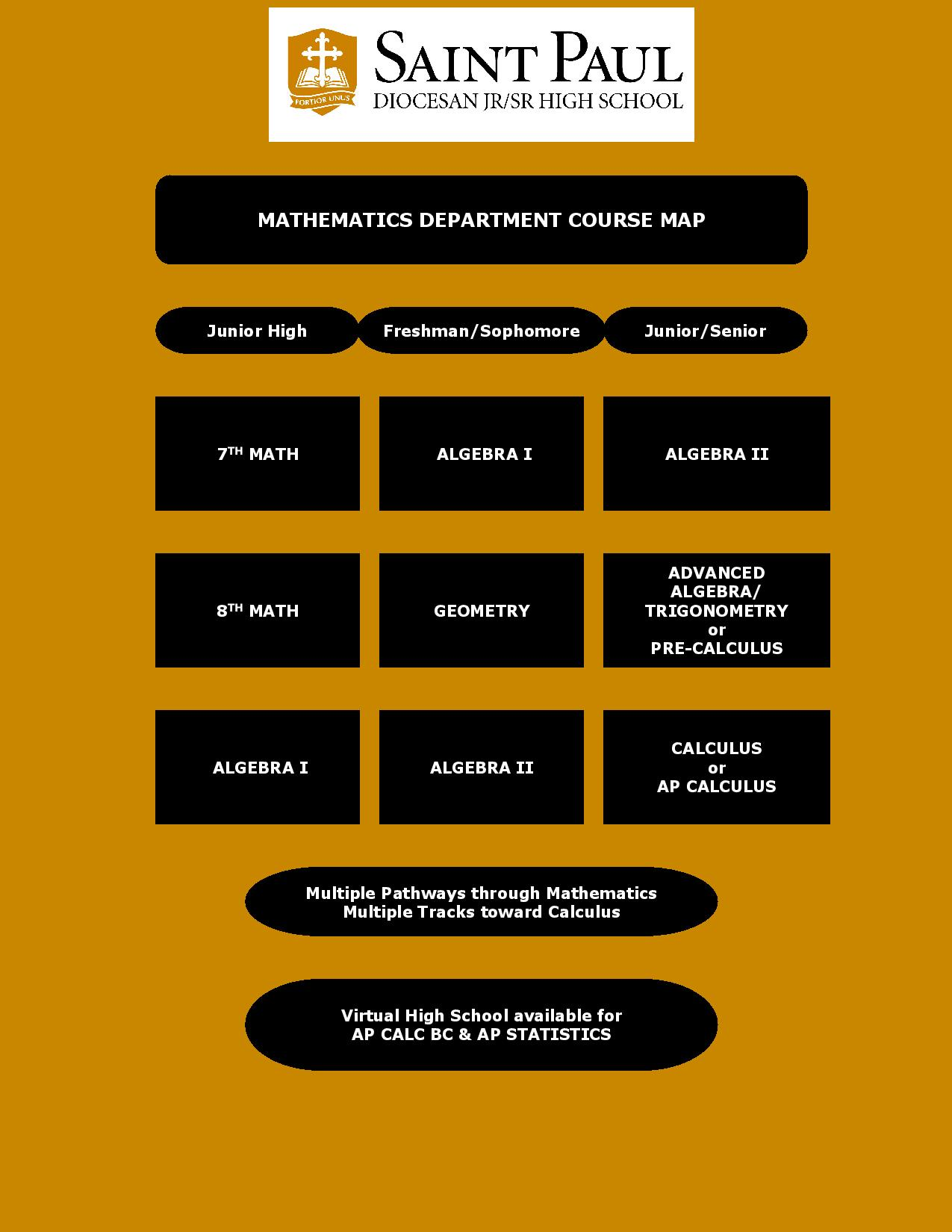Name TitleAmidon, Carole Math TeacherDecoteau, Thomas Mathematics Teacher/ Track CoachMarcotte, Gregory Mathematics Teacher/ Cross Country CoachPace, John Mathematics TeacherRusso, Susan Mathematics Department Chair
##### MATHEMATICS DEPARTMENT

Math 7 Full Year

The goal of this course is to introduce students to algebraic thinking. Grade 7 math focuses on developing the following skill-sets: applying proportional relationships, understanding of operations with rational numbers, working with linear expressions and equations, solving problems involving scale drawings and informal geometric constructions, working with 2- and 3-dimensional objects to solve problems involving area, surface area and volume, and drawing inferences about populations based on samples.

Math 8 Full Year

The purpose of this course is to advance the concrete skills learned in elementary school through the application of the more abstract mathematical concepts of high school mathematics. Grade 8 math topics of study include: formulating and reasoning about expressions and equations, including modeling an association two variables with a linear equation, solving linear equations and systems of linear equations, grasping the concept of a function and using functions to describe quantitative relationships, analyzing two- and three-dimensional space and figures using distance, angle, similarity, and congruence, and understanding and applying the Pythagorean Theorem. (NOTE: Upon teacher recommendation, a small number of students may be able to bypass 8th grade math and take Algebra I.)

Algebra I Grade 9 1 Credit

The primary goal of this course is to develop confidence and fluidity in dealing with algebraic expressions and equations. Algebra I extends the ideas formed in earlier courses with a focus on the following areas: analyzing relationships between quantities and reasoning with equations: writing, interpreting, and translating between various forms of linear equations and inequalities, and using them to solve problems: working with functions given by graphs, equations and tables, exploring systems of equations and inequalities, understanding integer exponents to consider exponential functions: extending the laws of exponents to rational exponents: operating with polynomials.

Math Seminar Grade 9 0.5 Credit

The purpose of this semester mathematics course is to provide foundational support to beginning high school students as they encounter a college preparatory mathematics curriculum with Algebra I. Topics of study include reinforcement of pre-Algebra mathematical concepts, variable manipulation, factorization, and rules of evaluation of expression. Taken as a companion course with Algebra I, student placement in Math Seminar comes at the recommendation of the department chair.

Geometry Grade 9 – 10 1 Credit

The purpose of this course is to develop students’ spatial and logical reasoning skills. This course involves primarily plane geometry with work in three dimensions. Topics of study include induction, deduction, elementary principles of logic, properties of lines, angles, polygons, circles, measurement (length, area, and volume), congruence, similarity, ratios and proportions. Also included are introductions to trigonometry, coordinate geometry, and formal proofs.

Algebra II Grade 10 – 11 1 Credit

The purpose of Algebra II is to build upon the foundations of Algebra I. Advancing their work with linear, quadratic, and exponential functions, students extend their understanding of functions to include polynomial, rational, and radical functions. Students work closely with the expressions that define the functions, and continue to expand their abilities to model situations and to solve equations, including solving quadratic equations over the set of complex numbers and solving exponential equations using the properties of logarithms. Trigonometry may be introduced.

Pre-Calculus Grade 11 – 12 1 Credit

This course is for those students seeking higher mathematics in preparation for Calculus. Topics will include exponential and logarithmic functions, rational equations, conic sections, trigonometry and trigonometric applications, inverse trigonometric functions, probability, statistics sequences and series including sigma notation, and the course will conclude with parametric equations and introduction to limits. Prerequisite: Successful completion of Algebra II (Honors: Accelerated College Prep).